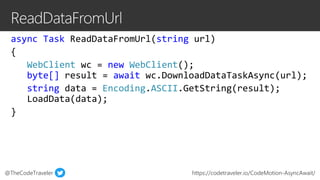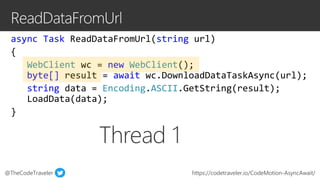Successfully reported this slideshow.×
1 of 27

Correcting Common Async/Await Mistakes in .NET

2

Share

Did you know that the .NET compiler turns our async methods into classes? And that .NET adds a try/catch block to each of these classes, potentially hiding thrown exceptions? It's true! In this session, we will learn how to best use async/await in C# by analyzing how .NET compiles our async code. Join me as we take an existing app and optimize its async code together, showing off performance gains, better exception handling, improved run-time speed, and smaller app size!

See all

See all

Correcting Common Async/Await Mistakes in .NET

1. 1. @TheCodeTraveler https://codetraveler.io/CodeMotion-AsyncAwait/
8. 8. @TheCodeTraveler https://codetraveler.io/CodeMotion-AsyncAwait/ async Task ReadDataFromUrl(string url) { WebClient wc = new WebClient(); byte[] result = await wc.DownloadDataTaskAsync(url); string data = Encoding.ASCII.GetString(result); LoadData(data); } private string <data>5_3; private byte[] <result>5_2; private WebClient <wc>5_1; public string url;
10. 10. @TheCodeTraveler https://codetraveler.io/CodeMotion-AsyncAwait/ public void MoveNext() { uint num = (uint)this.\$PC; this.\$PC = -1; try { switch (num) { case 0: this.<wc>__0 = new WebClient(); this.\$awaiter0 = this.<wc>__0.DownloadDataTaskAsync(this.url).GetAwaiter(); this.\$PC = 1; ... return; break; case 1: this.<result>__1 = this.\$awaiter0.GetResult(); this.<data>__2 = Encoding.ASCII.GetString(this.<result>__1); this.\$this.LoadData(this.<data>__2); break; default: return; } } catch (Exception exception) { ... } this.\$PC = -1; this.\$builder.SetResult(); }
11. 11. @TheCodeTraveler https://codetraveler.io/CodeMotion-AsyncAwait/ public void MoveNext() { uint num = (uint)this.\$PC; this.\$PC = -1; try { switch (num) { case 0: this.<wc>__0 = new WebClient(); this.\$awaiter0 = this.<wc>__0.DownloadDataTaskAsync(this.url).GetAwaiter(); this.\$PC = 1; ... return; break; case 1: this.<result>__1 = this.\$awaiter0.GetResult(); this.<data>__2 = Encoding.ASCII.GetString(this.<result>__1); this.\$this.LoadData(this.<data>__2); break; default: return; } } catch (Exception exception) { ... } this.\$PC = -1; this.\$builder.SetResult(); } case 0: this.<wc>__0 = new WebClient(); this.\$awaiter0 = this.<wc>__0.DownloadDataTaskAsync(this.url).GetAwaiter(); this.\$PC = 1; ... return;
12. 12. @TheCodeTraveler https://codetraveler.io/CodeMotion-AsyncAwait/ public void MoveNext() { uint num = (uint)this.\$PC; this.\$PC = -1; try { switch (num) { case 0: this.<wc>__0 = new WebClient(); this.\$awaiter0 = this.<wc>__0.DownloadDataTaskAsync(this.url).GetAwaiter(); this.\$PC = 1; ... return; break; case 1: this.<result>__1 = this.\$awaiter0.GetResult(); this.<data>__2 = Encoding.ASCII.GetString(this.<result>__1); this.\$this.LoadData(this.<data>__2); break; default: return; } } catch (Exception exception) { ... } this.\$PC = -1; this.\$builder.SetResult(); } case 1: this.<result>__1 = this.\$awaiter0.GetResult(); this.<data>__2 = Encoding.ASCII.GetString(this.<result>__1); this.\$this.LoadData(this.<data>__2); break;
13. 13. @TheCodeTraveler https://codetraveler.io/CodeMotion-AsyncAwait/ public void MoveNext() { uint num = (uint)this.\$PC; this.\$PC = -1; try { switch (num) { case 0: this.<wc>__0 = new WebClient(); this.\$awaiter0 = this.<wc>__0.DownloadDataTaskAsync(this.url).GetAwaiter(); this.\$PC = 1; ... return; break; case 1: this.<result>__1 = this.\$awaiter0.GetResult(); this.<data>__2 = Encoding.ASCII.GetString(this.<result>__1); this.\$this.LoadData(this.<data>__2); break; default: return; } } catch (Exception exception) { ... } this.\$PC = -1; this.\$builder.SetResult(); } try { catch (Exception exception) { . . . }
14. 14. @TheCodeTraveler https://codetraveler.io/CodeMotion-AsyncAwait
15. 15. @TheCodeTraveler https://codetraveler.io/CodeMotion-AsyncAwait
16. 16. @TheCodeTraveler https://codetraveler.io/CodeMotion-AsyncAwait/
17. 17. @TheCodeTraveler https://codetraveler.io/CodeMotion-AsyncAwait/
18. 18. @TheCodeTraveler https://codetraveler.io/CodeMotion-AsyncAwait/
19. 19. @TheCodeTraveler https://codetraveler.io/CodeMotion-AsyncAwait/
20. 20. @TheCodeTraveler https://codetraveler.io/CodeMotion-AsyncAwait/
21. 21. @TheCodeTraveler https://codetraveler.io/CodeMotion-AsyncAwait/
22. 22. @TheCodeTraveler https://codetraveler.io/CodeMotion-AsyncAwait https://codetraveler.io/CodeMotion-AsyncAwait/
23. 23. @TheCodeTraveler https://codetraveler.io/CodeMotion-AsyncAwait https://codetraveler.io/CodeMotion-AsyncAwait/

Editor's Notes

• Every time we add the `async` keyword, the compiler creates a new class.
Each class increases our app size by appx. 100 bytes
• Every time we add the `async` keyword, the compiler creates a new class.
Each class increases our app size by appx. 100 bytes
• Every time we add the `async` keyword, the compiler creates a new class.
Each class increases our app size by appx. 100 bytes